• 此提交实现了期望最大化算法并在简单的 2D 数据集上对其进行了测试。 期望最大化（EM）算法是一种迭代方法，用于在统计模型中依赖于未观察到的潜在变量的情况下，找到参数的最大似然或最大后验（MAP）估计。 EM 迭代...matlab
• 实现了期望最大化算法，用来聚类，隐藏变量假设为常数分布且相等，使用高斯混合模型。在iris数据集上测试，准确率约为80%。
• 文章的目录 一、最大期望算法简介 二、相关知识 2.1贝叶斯 2.2最大似然估计 2.3Jensen不等式 2.4高斯分布 三、EM算法 3.1实例理解 3.2EM算法求解步骤 3.3EM算法推导 3.4EM算法_python
• 它是期望最大化算法的一种实现，它带有数据集的完整图形和绘图。 无需指定复杂的属性，只需运行脚本即可享受！matlab
• 高斯混合模型的期望最大化算法的实现， 考虑 20 个点的数据并使用 EM 算法使用两个高斯分布对该数据进行建模matlab
• 基于半随机滤波-期望最大化算法的剩余寿命在线预测研究论文
• 最大似然期望最大化算法 分两个语言版本： Matlab:主函数为demo_MLEM_Simulation.m python: im = phantom(im_size, im_size)函数是Matlab内置的，生产一个im_size * im_size的矩阵（图片），在python中直接把矩阵...
• 该算法用期望最大化算法对初值和噪声的统计特性进行估计; 用求容积卡尔曼平滑器估计状态向量和未知参数。在期望最大化算法的求期望步骤中，所求的期望值通过求容积规则获得，用较少的采样点保证了估计精度; 在期望...研究论文
• K means segmentation method has an underlying assumption that each element cannot belong to two clusters at the same time. Sometimes, an element in transition area between two clusters are hard to ...
• 这是一些关于期望最大化算法的模拟和玩具示例，以及它在高斯混合模型和隐马尔可夫模型中的应用。 期望最大化 (EM) 期望最大化 (EM) 是一种简洁的算法，用于学习具有隐藏变量的概率模型，包括 HMM 和 GMM。 学习这些...R
• 这一章中，将EM算法进一步推广。为此先讲解了Jensen不等式，再由含有隐参数的分布，符合凸函数条件时可使用EM算法有效解决此类问题。
在上一章中，我们为了解决拟合混合高斯模型的拟合问题已经接触了EM算法。这一章里，我们会进一步扩展EM算法的应用，你会发现它可以用于解决一大类包含隐参数的估计问题。让我们从Jensen不等式开始我们的讨论。
1 Jensen 不等式
设

f
$f$是一个定义域为实数的函数，回忆前面的内容，当f′′(x)≥0$f^{"}(x) \ge 0$是函数

f
$f$就是一个凸函数（下凸）。而当f$f$的输入是一个向量时，当它的海森矩阵是一个半正定矩阵时，我们可以说函数

f
$f$是一个严格凸函数。Jensen不等式的表述如下：

定理. 设f$f$是一个凸函数，

X
$X$是一个随机变量。那么：

E[f(X)]≥f(EX).
E[f(X)] \ge f(EX).

不仅如此，若

f
$f$为严格凸时，那么E[f(X)]=f(EX)$E[f(X)] = f(EX)$当且仅当

X=E[X]
$X = E[X]$的概率为1时发生。关于定理的阐述我们可以看看下面这张图片：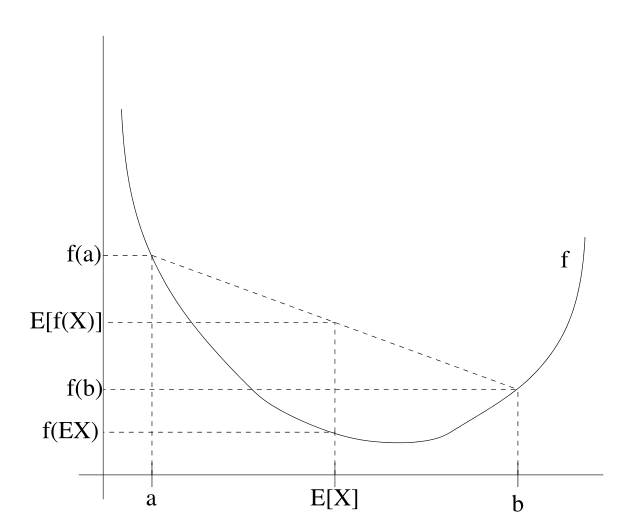图中凸函数f是实线绘制的曲线，随机变量

X
$X$有50%的概率是a，50%的概率是b，所以X$X$的期望是a,b的中点。从这个例子可以看到，只要

f
$f$是凸函数，必有E[f(X)]≥f(EX)$E[f(X)] \ge f(EX)$。

2 EM算法
设某估计问题中有m个独立的样本

{x(1),…,x(m)}
$\{ x^{(1)}, \ldots, x^{(m)} \}$。我们希望使模型

p(x,z)
$p(x,z)$的参数和数据拟合，则对数似然函数写成如下形式：

ℓ(θ)=∑i=1mlog p(x;θ)=∑i=1mlog∑zp(x,z;θ).

\begin{align}
\ell(\theta) &= \sum_{i=1}^m \text{log} \ p(x; \theta)\\
&= \sum_{i=1}^m \text{log} \sum_z p(x,z; \theta).\\
\end{align}

由于无法直接求解参数

θ
$\theta$的极大似然估计，引入隐参数

z(i)
$z^{(i)}$，如果假设隐参数的值已知，那么求解极大似然估计就会变得很容易。
这时求最大似然估计，EM算法是一个行之有效的方法。直接最大化

ℓ(θ)
$\ell(\theta)$很困难，但我们的策略是先构造

ℓ
$\ell$的下界(E步骤)，再最优化其下界(M步骤)。过程如下图所示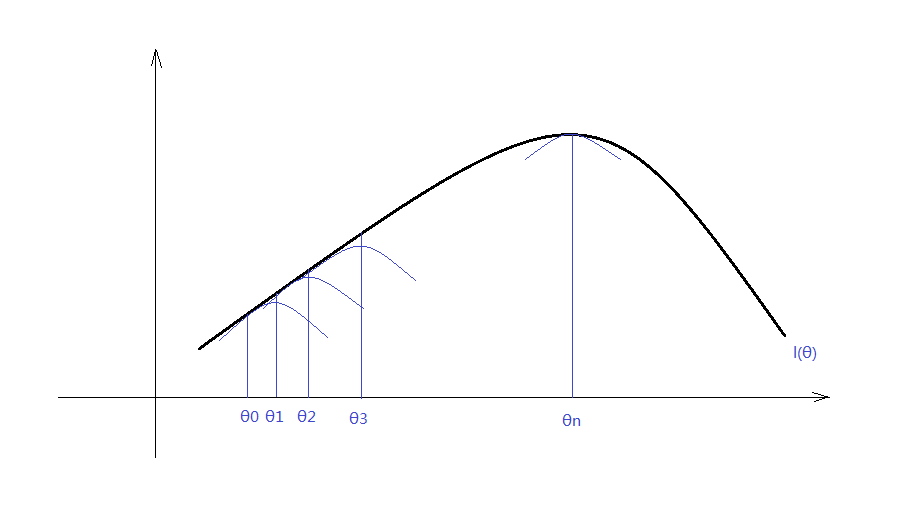对每一个

i
$i$，设z$z$服从

Qi
$Q_i$分布(

∑zQi(z)=1,Qi(z)≥0
$\sum_z Q_i(z) = 1, Q_i(z) \ge 0$)，则下式成立：

∑ilogp(x(i);θ)=∑ilog∑z(i)p(x(i),z(i);θ)=∑ilog∑z(i)Qi(z(i))p(x(i),z(i);θ)Qi(z(i))=∑ilog Ez(i)∼Qi[p(x(i),z(i);θ)Qi(z(i))]≥∑iEz(i)∼Qi [logp(x(i),z(i);θ)Qi(z(i))]≥∑i∑z(i)Qi(z(i)) logp(x(i),z(i);θ)Qi(z(i))(1)(2)(3)

\begin{align}
\sum_i \text{log} p(x^{(i)}; \theta) &= \sum_i \text{log} \sum_{z^{(i)}} p(x^{(i)}, z^{(i)}; \theta) &(1)\\
&= \sum_i \text{log} \ \text{E}_{z^{(i)} \sim Q_i} \left[ \frac{p(x^{(i)}, z^{(i)}; \theta)}{Q_i(z^{(i)})} \right] \\
&\ge \sum_i \text{E}_{z^{(i)} \sim Q_i} \ \left[\text{log} \frac{p(x^{(i)}, z^{(i)}; \theta)}{Q_i(z^{(i)})} \right]\\
&\ge \sum_i \sum_{z^{(i)}} Q_i(z^{(i)}) \ \text{log} \frac{p(x^{(i)}, z^{(i)}; \theta)}{Q_i(z^{(i)})} &(3)\\
\end{align}

因为

f′′(x)=−1/x2<0
$f^{"}(x) = -1/x^2 \lt 0$，

f(x)=log x
$f(x) = \text{log}\ x$是一个凹函数。第四步可以根据Jensen不等式求得。
对于任意的分布

Qi
$Q_i$，方程(3)给出了对数似然函数

ℓ(θ)
$\ell(\theta)$的下界。这时

Qi
$Q_i$分布有很多可能的选择，我们应该如何决定呢？如果我们现在有关于参数

θ
$\theta$的假设值，那么很自然下界的选择要和

θ
$\theta$相关。  要使下界的选择与

θ
$\theta$相关，我们需要推导中使用Jensen不等式的地方变为相等。为此期望值需要是一个常数变量。则有：

p(x(i),z(i);θ)Qi(z(i))=c

\frac{p(x^{(i)}, z^{(i)}; \theta)}{Q_i(z^{(i)})} = c

为使常数c不依赖

z(i)
$z^{(i)}$的取值。我们需要

Qi(z(i))
$Q_i(z^{(i)})$与

p(x(i),z(i);θ)
$p(x^{(i)}, z^{(i)}; \theta)$成比例。
实际上因为

∑zQi(z(i))=1
$\sum_z Q_i(z^{(i)}) = 1$，这进一步告诉我们：

Qi(z(i))=p(x(i),z(i);θ)∑zp(x(i),z(i);θ)=p(x(i),z(i);θ)p(x(i);θ)=p(z(i)|x(i);θ)

\begin{align}
Q_i(z^{(i)}) &= \frac{p(x^{(i)}, z^{(i)}; \theta)}{\sum_z p(x^{(i)}, z^{(i)}; \theta)} \\
&= \frac{p(x^{(i)}, z^{(i)}; \theta)}{p(x^{(i)}; \theta)} \\
&= p(z^{(i)} | x^{(i)}; \theta) \\
\end{align}

我们令

Qi
$Q_i$为给定

x(i)
$x^{(i)}$与参数

θ
$\theta$关于

z(i)
$z^{(i)}$的后验概率。
通过选择

Qi
$Q_i$，我们求对数似然函数的最大下界，这是E阶段。通过改变参数

θ
$\theta$，我们求方程(3)中的最大值。重复执行一上两个步骤就是EM算法：
循环至收敛 {
(E步骤)对每个i，令：

Qi(z(i))=p(z(i)|x(i);θ).

Q_i(z^{(i)}) = p(z^{(i)} | x^{(i)}; \theta).
(M步骤) 令：

θ:=arg maxθ∑i∑z(i)Qi(z(i)) logp(x(i),z(i);θ)Qi(z(i))

\theta := \text{arg}\  \max_\theta \sum_i \sum_{z^{(i)}} Q_i(z^{(i)}) \ \text{log} \frac{p(x^{(i)}, z^{(i)}; \theta)}{Q_i(z^{(i)})}

}  EM算法是一个一致收敛的算法。我们在算法描述时说循环至收敛。实际情况下判断收敛的方式一般为，当对数函数的增长小于某一设定值时，我们认为EM算法继续改善的能力已经很小了，即认为其收敛。
展开全文em算法 Jensen不等式
• EM算法是一种迭代算法，用于含有隐变量(hidden variable)或缺失数据(incomplete-data)的概率模型参数的极大似然估计。...每次迭代分为两步：E步，求期望；M步，求极大。概率模型有时既包含观测变量(observab...
EM算法是一种迭代算法，用于含有隐变量(hidden variable)或缺失数据(incomplete-data)的概率模型参数的极大似然估计。EM算法被广泛用于高斯混合模型(Guassian Mixture Model,GMM)和隐马尔可夫模型(Hidden Markov Model,HMM)的参数估计。每次迭代分为两步：E步，求期望；M步，求极大。概率模型有时既包含观测变量(observable variable)有包含隐变量。
作为机器学习十大算法之一，EM算法肯定有两把刷子。EM算法是基于极大似然估计(Maximum Likelihood Estimation,MLE)理论的优化算法，既然如此，在学习EM算法之前首先要对似然函数(Likelihood function)有个了解，对于似然函数，我也一直不是特别理解，通过查阅资料，对似然函数有了比较清晰的认识，既然EM算法是极大似然估计的一种扩展，那么我们先从极大似然估计说起。
目录
1 极大似然估计
1.1似然与概率
1.2 例子
2. EM算法
2.1 高斯混合模型(Gaussian misture model,GMM)
2.2 EM算法的原理
2.2.1 EM 算法的收敛性
3. EM算法的应用
参考文献

1 极大似然估计
引用维基百科里面的话说：似然函数是一种关于统计模型中的参数的函数，表示模型参数中的似然性。还是不太理解？只看这句话我也是云里雾里。我们来一点点的看。
1.1似然与概率
似然与概率有着千丝万缕的联系，在英语语境中，likelihood和probability是可以互换的，但是在数理统计中，这两个概念是不同的。“xxx的概率”中的xxx只能是概率空间中的事件；而“xxx的似然”中的xxx只能是参数。也就是说概率是随机变量的函数，而似然函数是参数的函数。
似然可以理解为条件概率的逆反。已知某个参数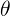时，事件X会发生的概率写作：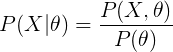由贝叶斯公式得：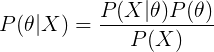因此，我们可以反过来构造表示似然性的方法：已知有事件X发生，运用似然函数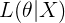，我们估计参数的可能性。在形式上，似然函数也是条件概率函数，但是我们关注的变量改变了。在贝叶斯公式中，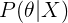表示后验概率，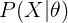表示似然，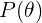表示先验概率，而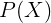是用于归一化的证据因子，给定X后，可以把它看做常量。
1.2 例子
以抛硬币为例，抛10次硬币，实验结果中有6次为“国徽”。我们假设以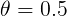为参数，实验结果的概率为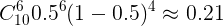；我们再假设以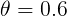为参数，实验结果的概率为：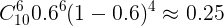，可以看到以作为参数时，抛10次硬币出现6次“国徽”的概率更大。
假设硬币的参数为，可以得到似然函数为：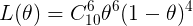,图像如下：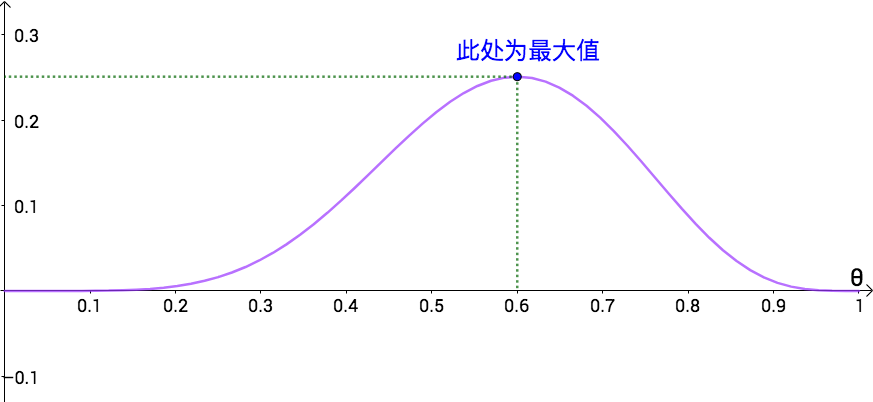可以看到似然函数在0.6处的取值最大，实际上似然函数就是推测的参数的分布。极大似然估计(Maximum likelihood estimation，MLE)是在做什么样的一件事呢，我们在知道事件发生(这里的事件就是抛10次硬币，出现6次“国徽”)的条件下，利用似然函数求出使似然函数最大的参数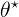,换句话说，极大似然估计能够找到模型的参数，使得观测发生的概率最大，反应到这个例子中就是，极大似然估计能找到模型参数，当时出现事件(抛10次硬币，出现6次“国徽”)的概率最大。
但是极大似然估计并不是能估算所有概率模型的参数，比如三硬币模型、高斯混合模型等等，因为在这些模型是由观测变量、隐变量以及参数共同决定的，接下来就以高斯混合模型为例子引入EM算法。
（三硬币模型）：假设有3枚硬币，分别记作A,B,C.这些硬币正面出现的概率分别为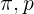和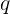。进行如下试验：先掷硬币A，根据其结果选出硬币B或硬币C，正面选硬币B，反面选硬币C；然后掷选出的硬币，掷硬币的结果，出现正面记作1，出现反面记作0；独立的重复n次试验(此处n = 10)，观测结果如下：  {1,1,0,1,0,0,1,0,1,1}。假设只能观测到掷硬币的结果，不能观测掷硬币的过程。那么该如何估计三硬币正面出现的概率，即三硬币模型的参数。
2. EM算法
2.1 高斯混合模型(Gaussian misture model,GMM)
高斯分布也称作正态分布，是我们生活中最常见的概率分布。我们的身高在样本数足够多的情况下就是服从高斯分布的，假设我们统计500个人(随机选取)的身高，并将身高数据绘制成直方图，如下图所示。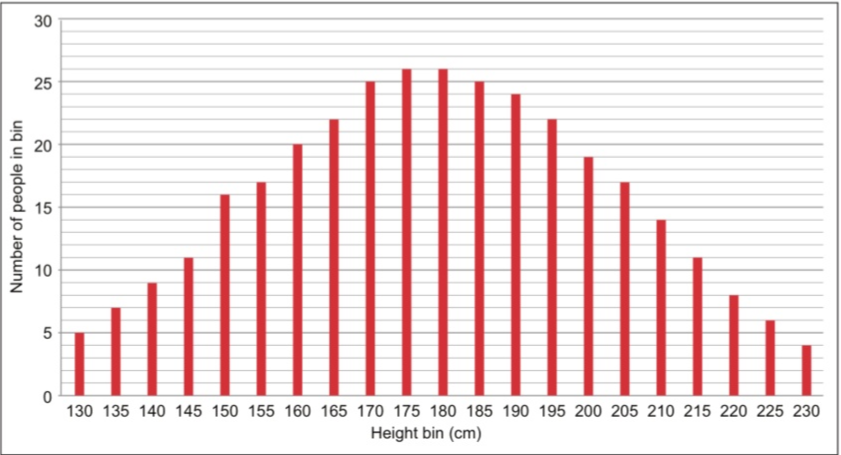我们可以认为身高数据是由上面这样的高斯分布随机生成的，如果考虑到男女，那么身高数据绘制成的直方图会变成下面这种形式：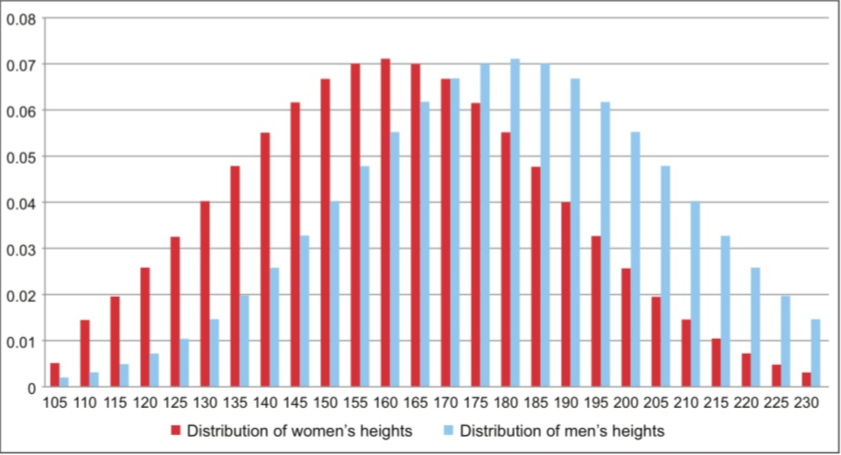这时候，身高数据是由两个不同的高斯模型共同生成的，也就是高斯混合模型如果随机给我们一个人身高数据，让我们判断这个人的性别，比如180cm，我们可以从上面的图中看到，180cm的数据由男性的高斯分布产生的概率更大一些，所以我们可以认为这个人是男性。这其实就是高斯混合模型可以用来聚类的基本思想。
(高斯混合模型)：高斯混合模型是指具有如下形式的概率分布模型：
(2.1)
其中，是第k个高斯模型的系数，也可以看做是权重，，，是高斯分布密度，。在男女身高这个例子里，。
假设观测数据单个高斯模型生成的，可以直接用极大似然估计来计算参数：
(2.2)
其中，我们可以直接对对数似然函数求偏导让偏导数为0，求得参数。
假设观测数据是由高斯混合模型生成的，此时的参数为,这时候，就有
(2.3)
此时的对数似然函数是远比单高斯模型的复杂，而且log函数里面还包含求和，这就比较复杂了，如果还是按照对对数似然函数求偏导的方法估计参数，可以实现，但是是相当困难的，方法总是比问题多，我们这是可以通过迭代的方法求解，这就类似于非概率模型中最小化平方误差函数时使用的梯度下降法，这种算法就是EM算法。
2.2 EM算法的原理
在高斯混合模型中观测数据是由高斯模型产生的，但是是由哪一个高斯模型产生的我们是不知道的，我们引入隐变量Z，对于EM算法我们有如下定义
(2.4)
当连续两次迭代和的差小于任意小的正数时，停止迭代，即时停止迭代。
给本来就复杂的式子再另外引入隐变量Z，这能解决问题？答案是肯定的，因为隐变量可以告诉我们观测数据是由哪个模型产生，这样的话我就相当于把某个数据择(zhai)出来，这样就回到了单个高斯模型的求参问题。但是加入隐变量有一个要求就是边缘分布不能改变，用式子表示是：
(2.5)
这里的是离散分布，所以用求和符号得到边缘分布，如果是连续分布，则需要积分，而高斯混合模型中的权重就是的先验。
2.2.1 EM 算法的收敛性
“走得太远了，不要忘记我们为什么要出发”。我们训练概率模型的目的就是求使对数似然函数最大的参数,而EM算法是通过迭代逐步近似的极大值的，也就是说那么我们怎么保证每一次迭代对应的对数似然函数值都会比上一次迭代对应的对数似然函数值要大呢。接下来我们证明一下。
对数似然函数可以写成下面的式子：
(2.6)
对上式等式两边分别以为分布对函数求期望得：
(2.7)
等式左边为：                                                                                     (2.8)
将式(2.8)代入(2.7)并式(2.7)等号右边展开得：
(2.9)
分别令:
(2.10)
(2.11)
其中中的第一个变量表示要极大化的参数，第二个变量表示参数的当前估计值。而且这个就是EM算法定义中要最大化的项，那么比较两个数值大小的方法通常有两种，一种是做差，一种是做商，这里我们采用做差的方法来验证。对上式中的分别取和并相减得到：
(2.12)
由式(2.4)知道，由于是使最大化的值，所以。对于第二项，有：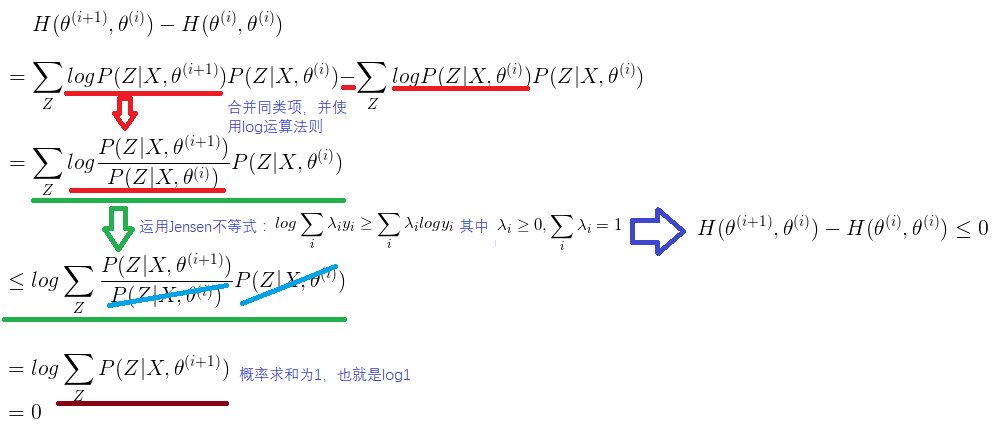所以就得到了：，这样就证明了每一次迭代比上一次迭代得到的对数似然函数要大，这样EM算法才能收敛。
关于EM算法有几点需要说明：
1、因为EM算法是一种迭代算法，所以需要给参数设置初值，初值的选择是随意的，但是EM算法对初值是敏感的，究竟选择怎么样的初值使模型训练结果最好，这需要不断的试
2、前面说到，EM算法中，E步是求期望，那么求得是谁的期望呢。求得是联合分布的对数似然函数以为分布的期望，即，把它定义为。
3、M步就是求期望的最大值，也就是的最大值，得到，这样就完成一次迭代。
4、当连续两次迭代和的差小于任意小的正数时，停止迭代，即时停止迭代。
3. EM算法的应用
以三硬币模型为例，使用EM算法求解模型参数。为了方便阅读，把三硬币模型的描述搬下来。
（三硬币模型）：假设有3枚硬币，分别记作A,B,C.这些硬币正面出现的概率分别为和。进行如下试验：先掷硬币A，根据其结果选出硬币B或硬币C，正面选硬币B，反面选硬币C；然后掷选出的硬币，掷硬币的结果，出现正面记作1，出现反面记作0；独立的重复n次试验(此处n = 10)，观测结果如下：  {1,1,0,1,0,0,1,0,1,1}。假设只能观测到掷硬币的结果，不能观测掷硬币的过程。那么该如何估计三硬币正面出现的概率，即三硬币模型的参数。
E步：

计算在模型参数下观测数据来自掷硬币B的概率

M步：
计算模型参数的新估计值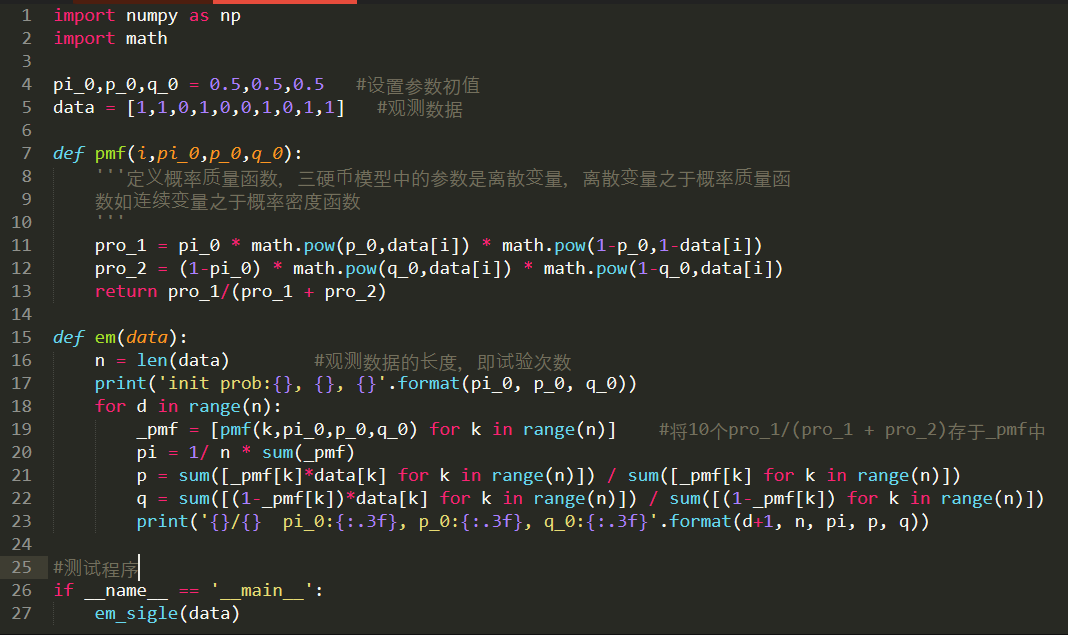参考文献
1. 统计学习方法                李航
2. 维基百科《似然函数 》      https://zh.wikipedia.org/wiki/%E4%BC%BC%E7%84%B6%E5%87%BD%E6%95%B0
3. 如何理解似然函数              https://www.zhihu.com/question/54082000
4. 如何通俗的理解“最大似然估计法”      https://mp.weixin.qq.com/s/SgngqSu4nOslI_zw4vS8Vg
5. 徐亦达自频道           http://i.youku.com/i/UMzIzNDgxNTg5Ng==?spm=a2hzp.8253869.0.0
6. 一文详解高斯混合模型原理           https://zhuanlan.zhihu.com/p/31103654
展开全文高斯混合模型 似然函数
• 该包演示了如何使用期望最大化算法来解决简化的一维多对象跟踪问题。 多目标跟踪通常涉及随着时间的推移进行一系列位置测量，然后回答两个问题：a.) 为了合理解释所观察到的所有位置测量，所需的最小目标数量是多少...matlab
• 该代码在Matlab中实现了期望最大化算法 使用EM_main.m运行EM代码 可以通过“ cluster_data”变量提供用户群集数据，也可以使用代码中提供的综合数据进行测试。 使用变量“中心”（Centers）更改要分类为数据的中心的...
• 1、极大似然和EM算法 ...在文本分类中有个极其强大的技术：期望最大化算法。 文本的自收敛分类： 期望最大化和收敛的必然性： 我们的目的是相近的点都被聚集到一个类别。同一类中各个点到中心的平...
1、极大似然和EM算法
2、EM算法存在的意义是什么？

前面多次介绍到文本分类，一是互联网各方面都会用到这些技术，另一方面这些技术几乎可以用到所用分类中。如用户分类、词分类、商品分类、甚至生物特征和基因分类等。在文本分类中有个极其强大的技术：期望最大化算法。
文本的自收敛分类：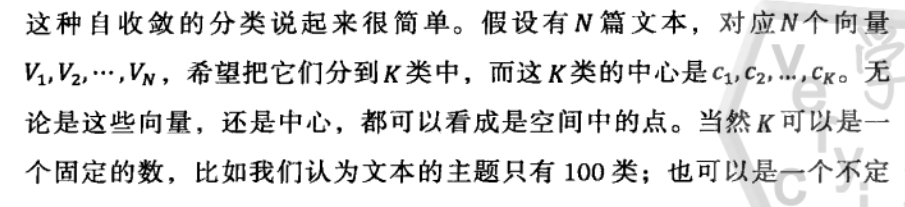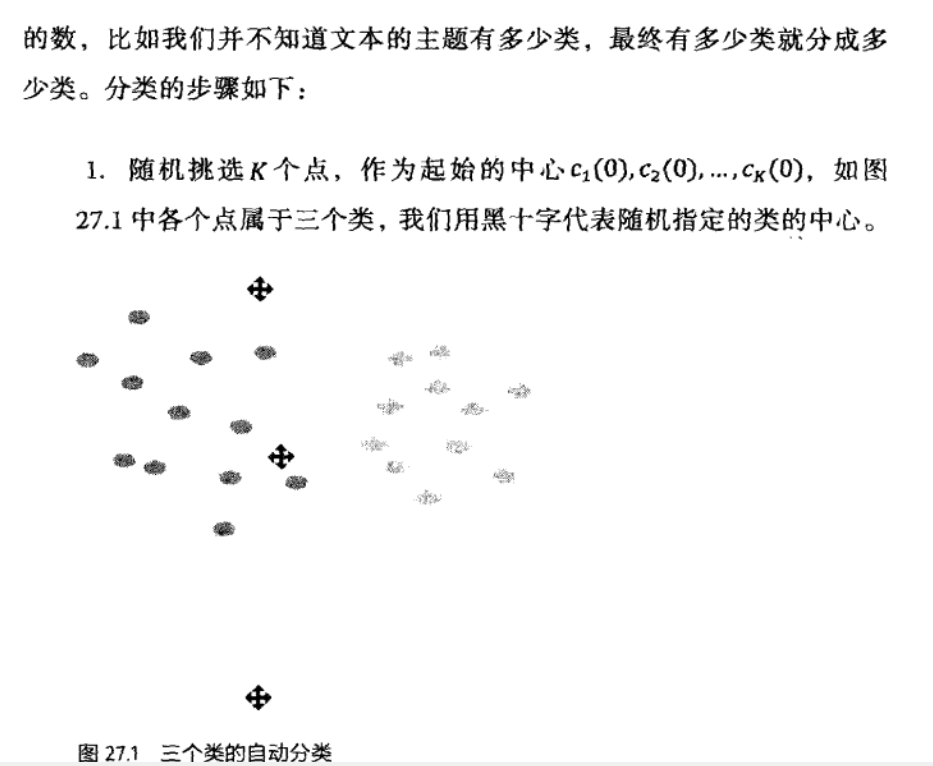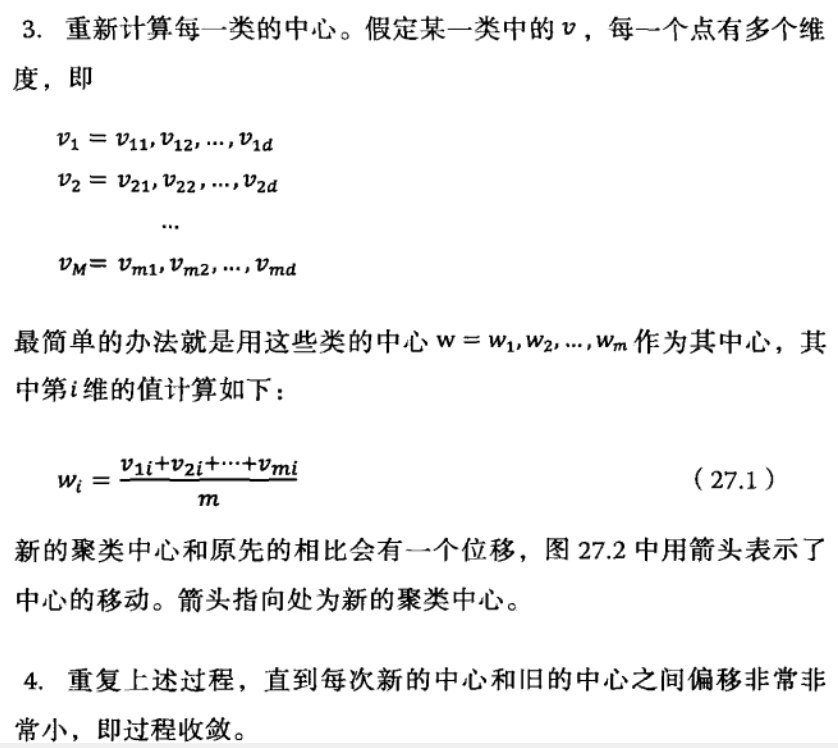期望最大化和收敛的必然性：
我们的目的是相近的点都被聚集到一个类别。同一类中各个点到中心的平均距离d较小，而不同类别间的中心的平均距离D较大。我们希望每一个迭代都会d比以前小，而D比以前大。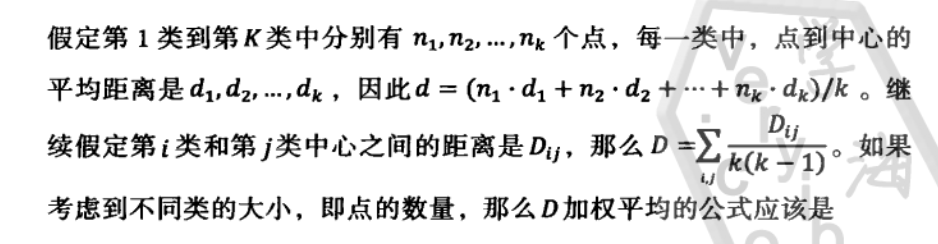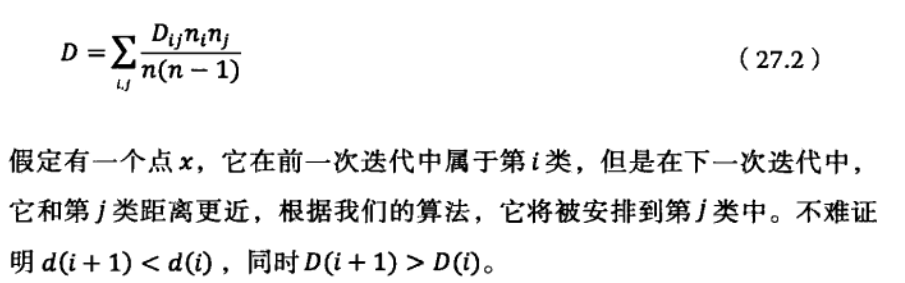将上面思想推广至一般机器学习，实际上包括两个过程和一个目标函数。
1、根据现有聚类结果，对所有数据点进行重新划分。
2、根据重新划分结果，得到新的聚类。
3、整个流程最大化目标就是点到聚类中心距离d和类间距离D。
首先计算各个观测数值输入到模型的结果，这过程叫做期望值计算过程；然后重新计算模型以最大化期望值成为最大化过程。这类算法统称为EM算法（Expectation Maximization）。
前面介绍到的很多算法其实都是EM算法。如隐马尔可夫模型中的训练算法Baum-Welch，E过程根据现有模型计算每个状态之间转移次数和每个状态的输出次数，M过程就是根据这些次数重新估算隐马尔科夫模型的参数。其最大化目标函数是观测值的概率。以及最大熵模型的训练算法GIS算法，E过程利用现有模型计算每个特征的数学期望值，M过程就是根据这些特征的数学期望和实际观测值的比值，调整模型参数，最大化目标函数是熵函数。
另外EM算法是否能够获得全局最优解？如果我们的目标函数是凸函数，那么一定可以获得全局最优解。而我们的熵函数是凸函数，如果在N维空间使用欧式距离做度量，聚类中我们试图优化的两个函数也是凸函数。但很多情况，如文本分类中的余弦距离不能保证是凸函数，因此EM算法有可能给出局部最佳解。吴军大牛称之为上帝算法。
展开全文• 期望最大化算法 (EM) 被广泛用于查找高斯概率密度函数 (pdf) 或在最大似然意义上拟合样本测量向量的简单高斯分量的混合参数 。 在我们的工作中，通过三个统计测试改进了用于高斯混合建模的期望最大化 (EM) 算法：...matlab
• matlab开发-期望最大化算法，以避免不合理的个人交易和分配。双变量正态反高斯分布的期望最大化算法
• 本算法根据《数据挖掘概念与技术》第三版（韩家炜）中的期望最大化算法（Expectation Maximum, EM）的步骤来实现的，EM算法属于基于概率模型的聚类的一种，它的主要步骤分为两步： 期望步：给定当前的簇中心，每个...
本算法根据《数据挖掘概念与技术》第三版（韩家炜）中的期望最大化算法（Expectation Maximum, EM）的步骤来实现的，EM算法属于基于概率模型的聚类的一种，它的主要步骤分为两步：
期望步：给定当前的簇中心，每个对象都被指派到簇中心离该对象最近的簇。：给定当前的簇中心，每个对象都被指派到簇中心离该对象最近的簇。
最大化步：给定簇指派，对于每个簇，算法调整其中心，使得指派到该簇的对象到该新中心的距离之和最小化。：给定簇指派，对于每个簇，算法调整其中心，使得指派到该簇的对象到该新中心的距离之和最小
主函数如下：
%%%% 采用期望最大化进行模糊聚类 ，EM
clc;
clear;
%加载文件
fileID = fopen('D:\matlabFile\EM\EM.txt');
DS=textscan(fileID,'%f %f');
fclose(fileID);
%将数据转换为矩阵格式
Dataset=cat(2,DS{1},DS{2});
%选择簇中心的点数量
p=2;
if p<size(Dataset,1)
% 任意选择聚类中心点
c_id=randperm(size(Dataset,1));
c_id=c_id(1:p);
%    c_id=[1 2];
% 初始化p的中心点
centers=Dataset(c_id',:);
% 限定最小值
err=0.005;
flag=1;
d1=0;
%簇中心收敛计算
while flag
% 计算隶属度矩阵
M=MembershipDegree(centers,Dataset);
% 计算新的中心点
centers_new=Maximization(M,Dataset);
d2=0;
for i=1:size(centers_new,1)
d2=d2+sqrt((centers_new(i,1)-centers(i,1))^2+(centers_new(i,2)-centers(i,2))^2);
end
if abs(d1-d2)<err
flag=0;
else
d1=d2;
centers=centers_new;
end
end
end
%显示簇中心
centers
MembershipDegree函数如下：
function result=MembershipDegree(centers,points)
M=zeros(1,size(centers,1));
for i=1:size(points,1)
point=points(i,:);
flag=0;
for j=1:size(centers,1)
center=centers(j,:);
if isequal(point,center)
flag=1;
break;
end
end
mm=[];
if flag
mm=zeros(1,size(centers,1));
mm(1,j)=1;
else
for j=1:size(centers,1)
center=centers(j,:);
mm(1,j)=(center(1,1)-point(1,1))^2+(center(1,2)-point(1,2))^2;
end
mm=mm/sum(mm);
end
M(i,:)=mm;
end
result=M;
end
Maximization函数如下：

function result=Maximization(M,points)
centers=zeros(1,2);
for i=1:size(M,2)
a=sum(M(:,i).^2);
b=M(:,i).^2;
x=sum(b.*points(:,1))/a;
y=sum(b.*points(:,2))/a;
centers(i,:)=[x,y];
end
result=centers;
end
测试数据如下，请保存为EM.txt
3 3
4 10
9 6
14 8
18 11
21 7

展开全文•研究论文
• 基于期望最大化算法的Rayleigh-Pearson混合模型参数估计研究论文
• 该项目在丢失数据的情况下实现基于期望最大化（EM）算法的回归模型开源软件
•研究论文
• HMRF的MATLAB实现，如“通过隐马尔可夫随机场模型和期望最大化算法对脑MR图像进行分段”（Zhang等人，2001年）所述。 HMRF被应用于从OASIS脑截面数据集中分割图像，但是提供的代码可以针对任何3D图像分割进行修改。 ...
• 实现期望最大化算法EM，对混合模型进行参数估计，得到参数的具体值。
• 期望最大化算法解决掷硬币问题。 C/C++语言，MFC做界面。
• ## EM-期望最大化算法

千次阅读 2015-11-04 21:01:55
最大期望算法（Expectation Maximization Algorithm，又译期望最大化算法），是一种迭代算法，用于含有隐变量（hidden variable）的概率参数模型的最大似然估计或极大后验概率估计。 中文名 em算法 外文名 ...
• ## 最大化期望算法（EM）详解

万次阅读 多人点赞 2018-07-30 09:15:53
我们知道最大似然估计的根本目的是根据抽样的到的样本（即数据），反推出最有...这个时候就要依靠最大化期望（EM）算法了。 简单的说，EM算法是在依赖于无法观测的隐藏变量的概率模型中，寻找参数最大似然估计或者...最大似然估计 凸函数 Jensen不等式
• em算法代码matlab实现期望最大化 Matlab中的期望最大化（EM）算法 此代码实现了Expectation-Maximization（EM）算法，并在简单的2D数据集上对其进行了测试。 期望最大化（EM）算法是一种迭代方法，用于在统计模型中......